Tangent to a circle – Circles | Class 10 Maths

• Last Updated : 24 Oct, 2021

Tangent to a Circle is a straight line that touches the circle at any one point or only one point to the circle, that point is called tangency. At the tangency point, the tangent of the circle will be perpendicular to the radius of the circle. In the below figure PQ is the tangent to the circle and a circle can have infinite tangents.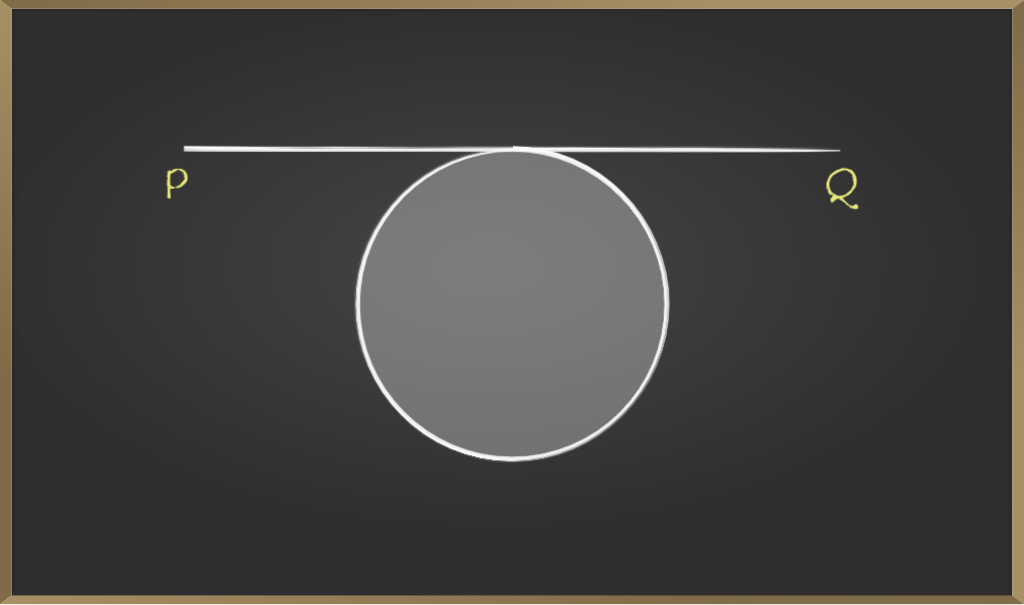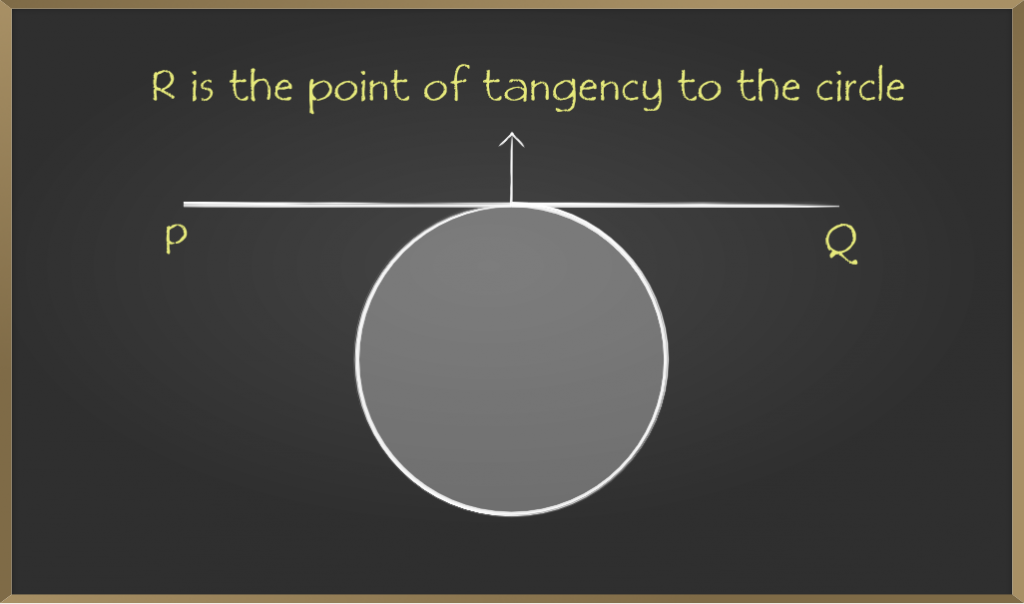• From the above figures, PQ is the tangent.
• The two tangents can be drawn parallel to a secant that can be drawn at a circle

Note: A circle can have an infinite number of tangents.

A tangent can be drawn between two circles in two ways. They are

Externally

An external tangent can be drawn between two circles in one way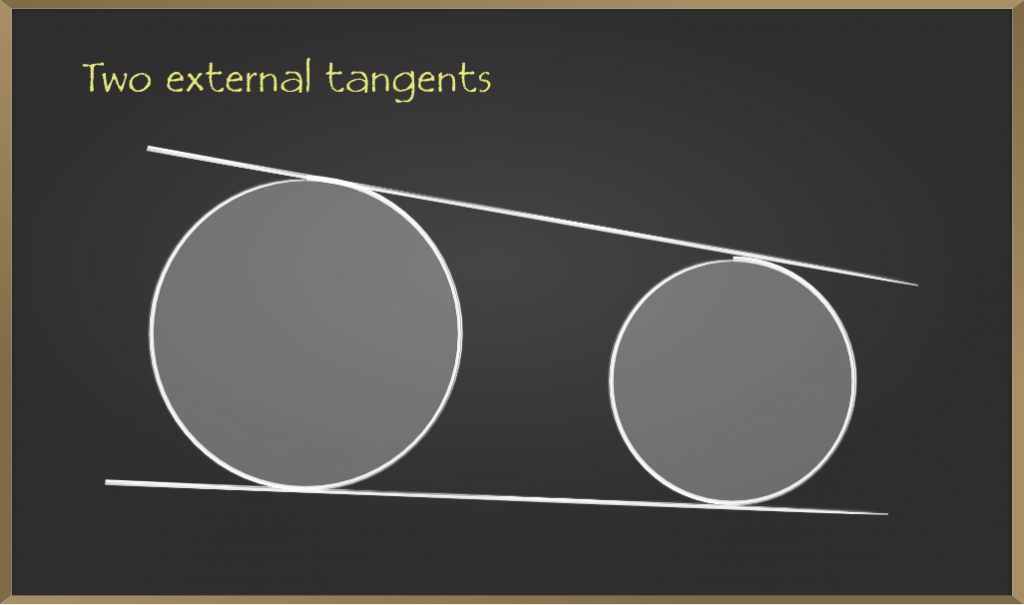• We can name the upper tangent as AB
• And lower tangent as CD

Example: AB is the common tangent to O, P circles. Find the length of AB.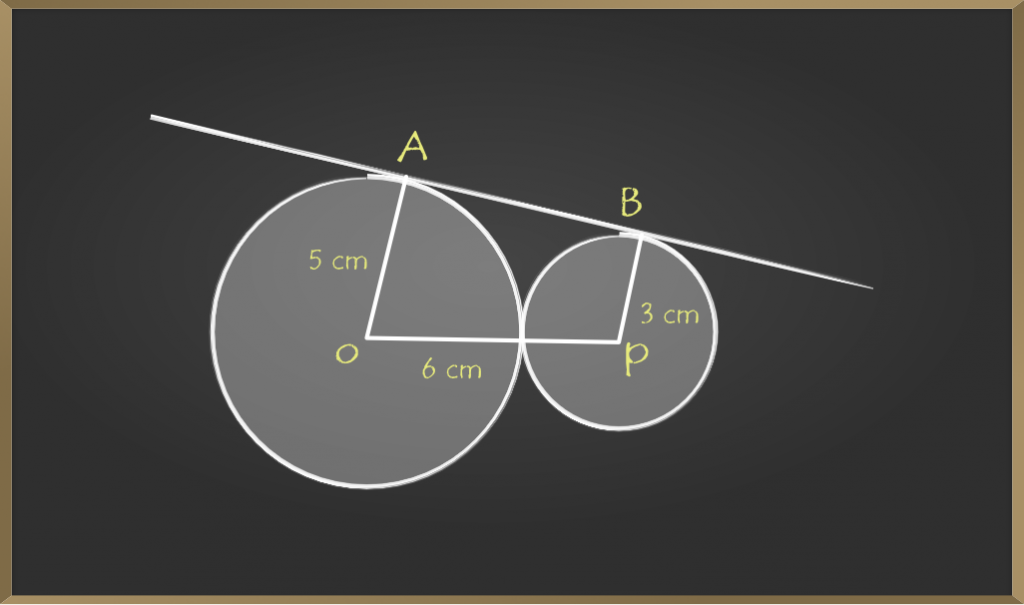Solution:

AB is a tangent,
Draw a line parallel to AB as shown below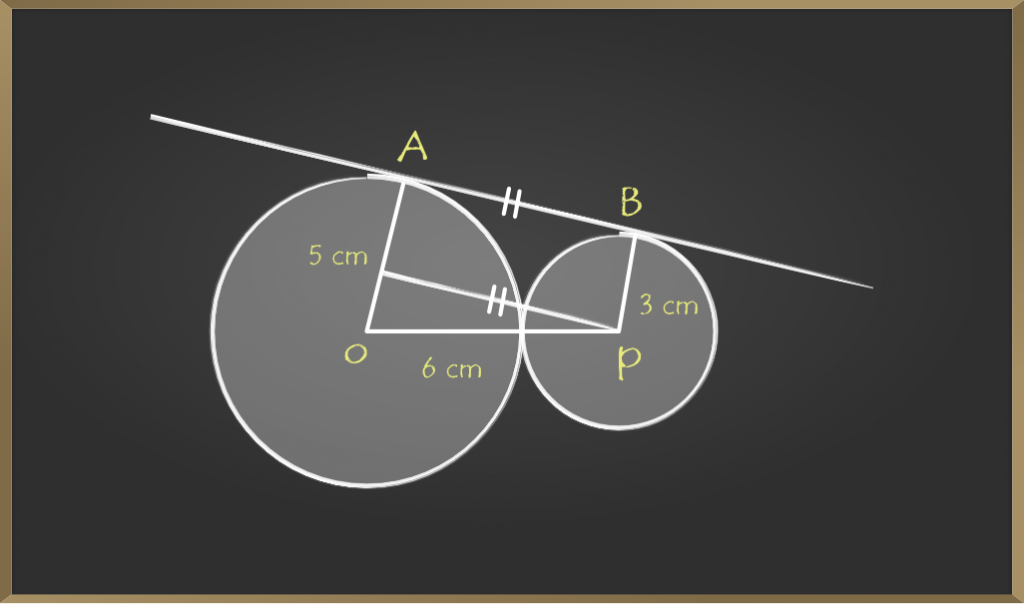Point on OA can be assumed as Q

Now POQ forms right angle triangle as shown below
So, 12 + B2 = 32
B2 = 9 – 1
B2 = 8
B = √8 cm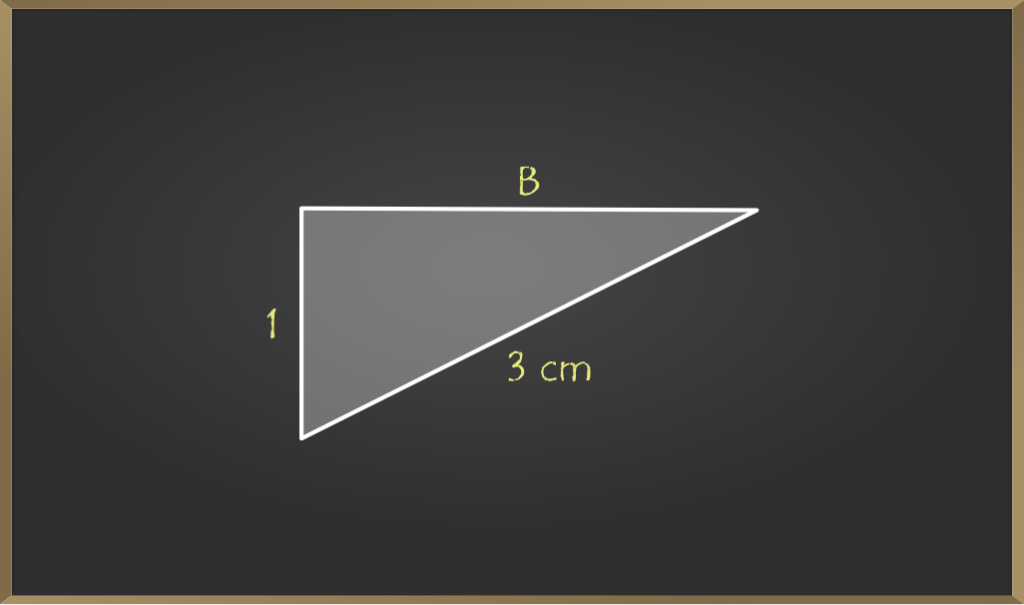so the length of AB is 2√8 cm

Internally

If Tangents of two circles intersect at a common point is called the internal tangents. We have four cases for internal tangents

Case 1

If the two circles touch at just one point, with one inside the other, there is just one line that is a tangent to both.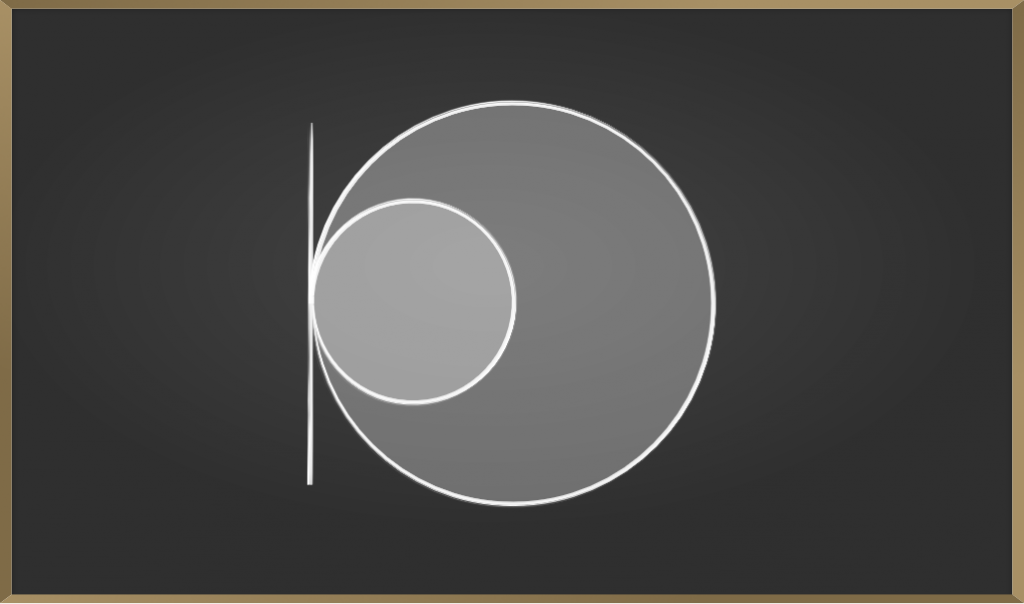Example: If The radius of the big circle is 6 cm and the small circle is 3 cm then find the shortest perpendicular distance from the common tangent to 2 circles.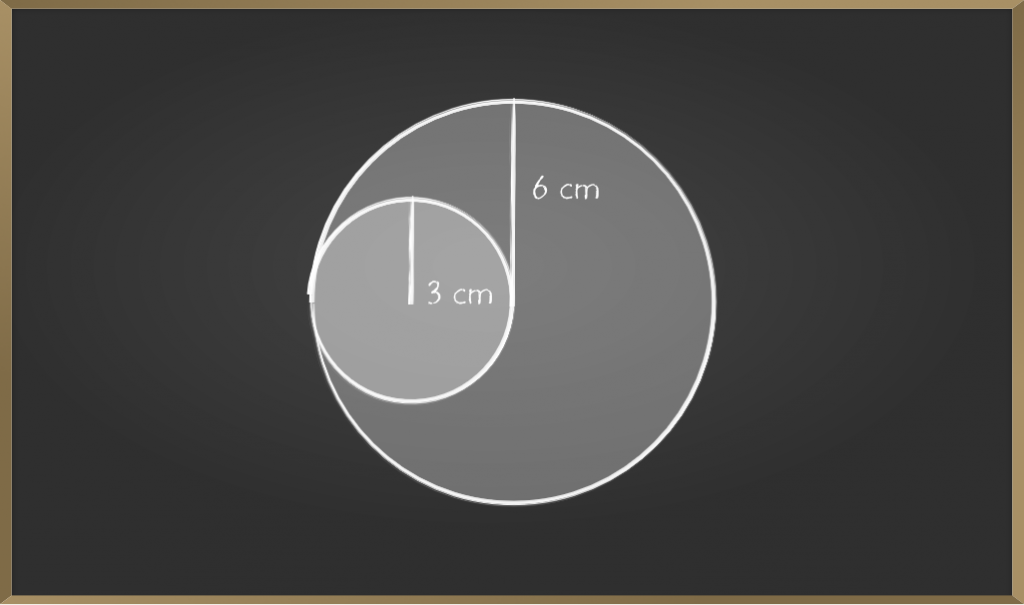Solution:

Given r1 = 6cm, r2 = 3cm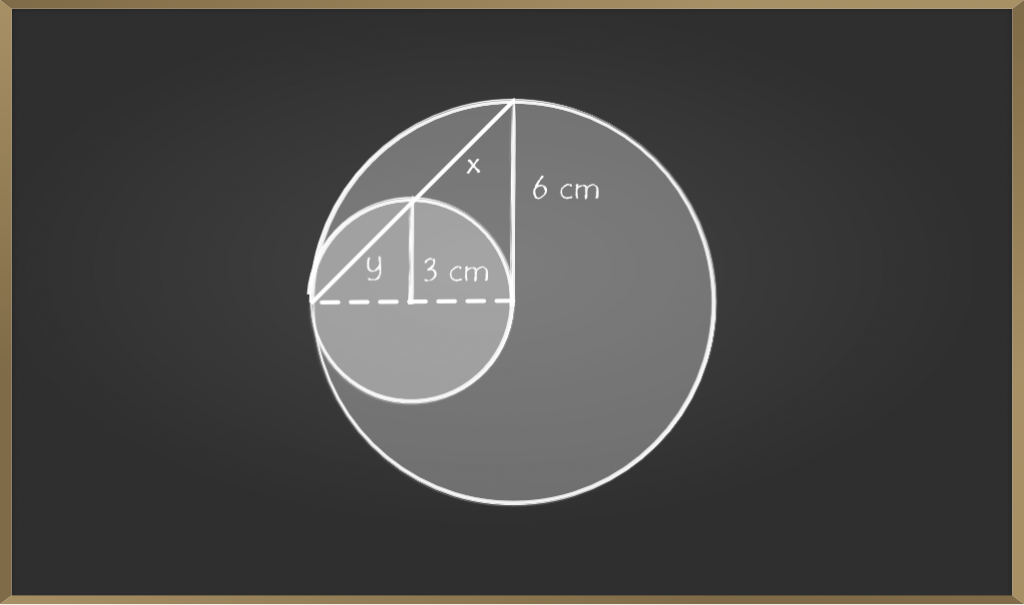For the big circle,
the shortest distance is

y2 = 32 + 32

y2 = 9 + 9

y = √18

for small circle, the shortest distance is

x2 = 62 + 6

x2 = 36 + 36

x = √72

Hence, the shortest distance from the tangent where it grazes and to perpendicular to top of the circle.

Case 2

A tangent of two circles is a common internal tangent. The intersection of the tangent and the line segment joining the centers is not empty.

For example, line AB common internal tangents.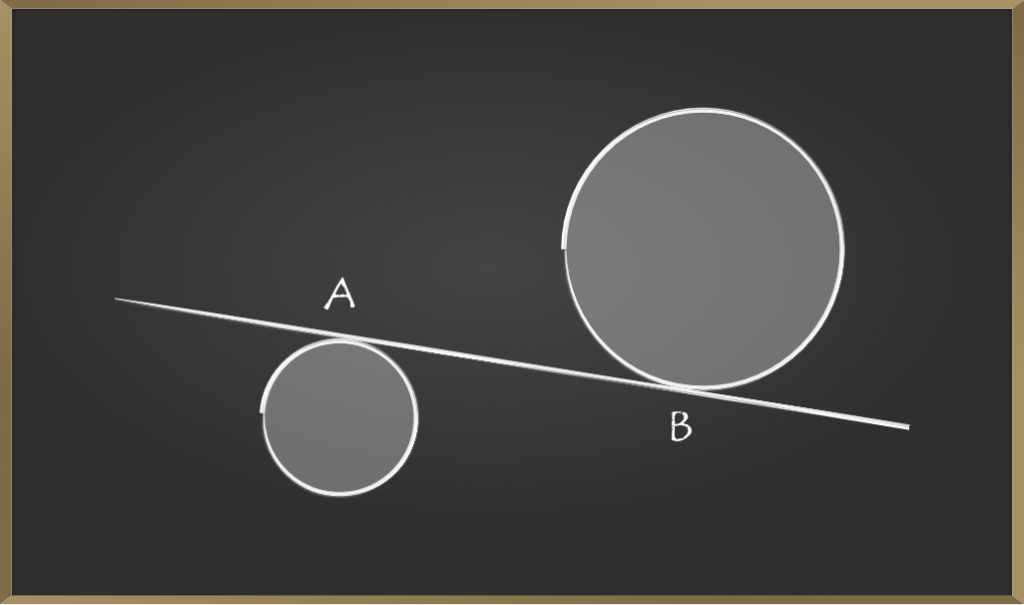Example: Find the number of common tangents to the circles x2 + y2 − 4x − 6y − 12 = 0 and x2 + y2 + 6x + 18y + 26 = 0.Solution:

Small circle equation is x2 + y2 − 4x − 6y − 12 = 0 and big circle equation is x2 + y2 + 6x + 18y + 26 = 0.

Centers of circles are C1 (2, 3) and C2 (−3, −9) and their radii are r1 = 5 and r2 = 8 Obviously r1 + r2 = C1C2 i.e., circles touch each other externally.

Hence, there are three common tangents.

Case 3

In case the tangents of two circles will intersect at a point we can name as O

• AB is the common tangent
• CD also a common tangent
• These two tangents AB, CD intersecting at one point.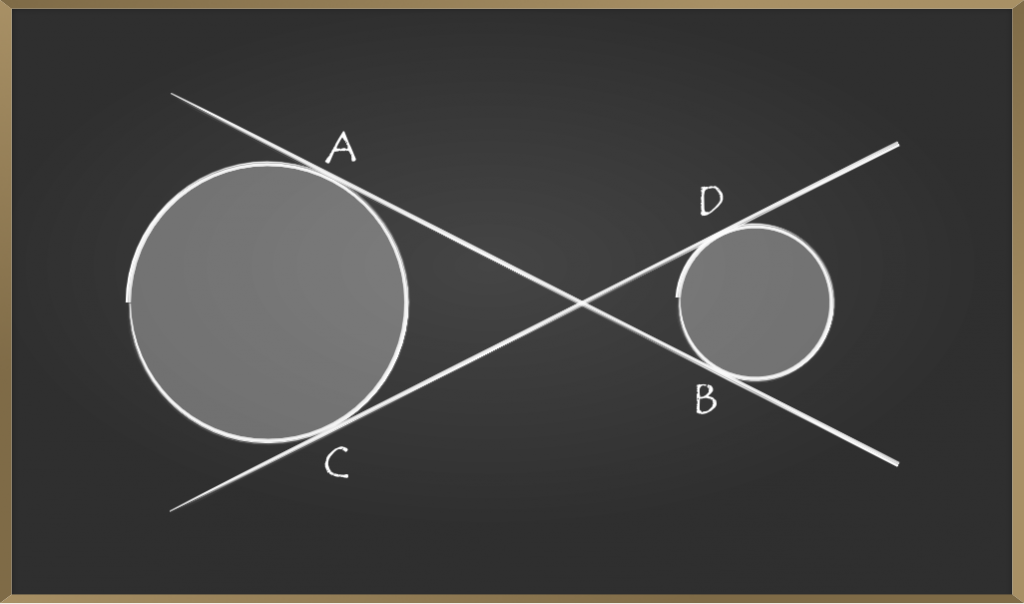Example: Given equations of 2 tangents with equations x + 2y + 1 = 0 and 2x + 3y + 5 = 0. Check whether the tangents will
intersect or not?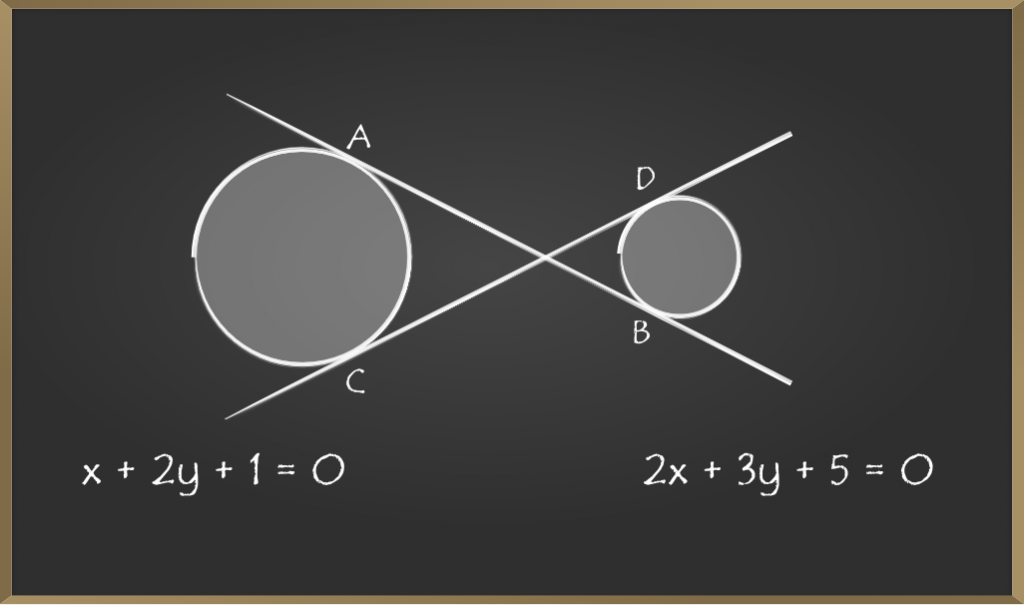Solution:

Firstly checking the slopes of two tangents.

1st equation = slope = – y/x = -2 /1

2nd equation = slope = – y/x = -3 /2

Hence there are no slopes, so the tangents will intersect.

Note 1: The set of circles cannot have common internal and external tangents.

Note 2: If one circle is inside another circle, then we cannot draw a tangent. It was shown below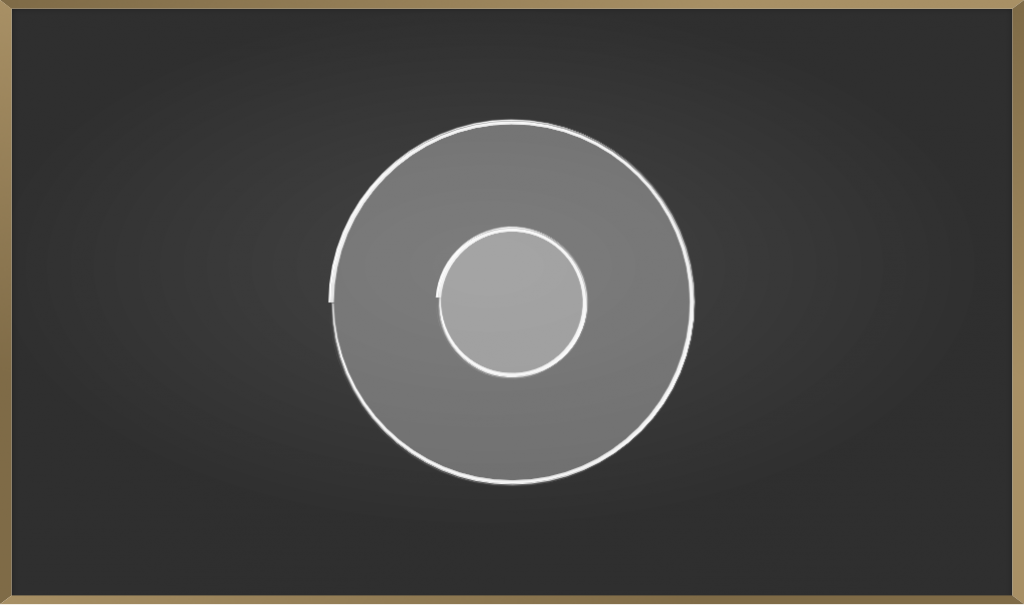Secant

The line which intersects two points on the circle is known as the secant. (or) The line which cuts the circle at two distinct points is called Secant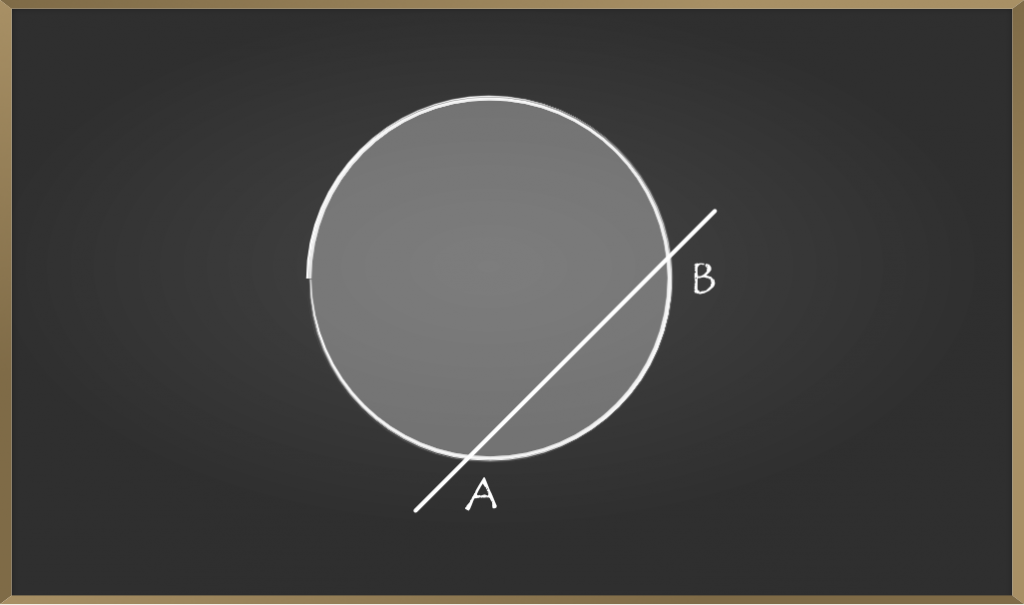• From the above figure, AB is the secant to the circle
• In the above figure the points A and B, two distinct points cutting the circle.
• The secant cut the circle in any direction.
• The secant can even be drawn from outside the circle.

Example 1: Describe the tangents and secants from the given figure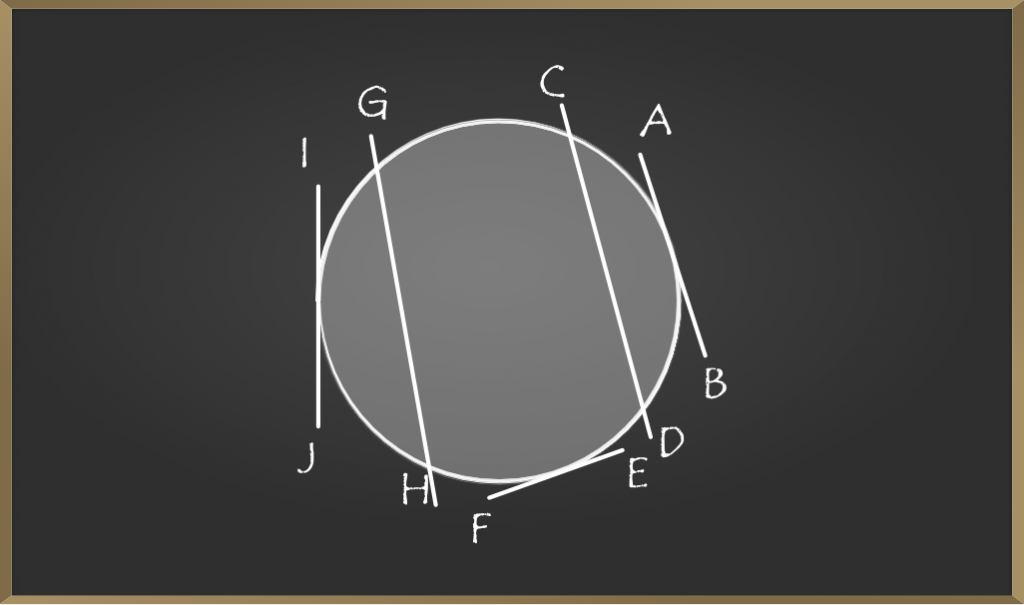Solution:

According to the above figure,

AB is a tangent.

CD is the secant.

EF is the tangent.

GH is the secant.

IJ is the tangent

Example 2: List out the number of tangents and secants from the given figureSolution:

From the figure,

we have 3 tangents, i.e AB, EF, IJ.

And we have 2 secants, i.e CD, GH.

Chord

The Line which divides a circle into two halves is called a chord. (or) The two distinct points which divide the circle into two equal parts called as chord.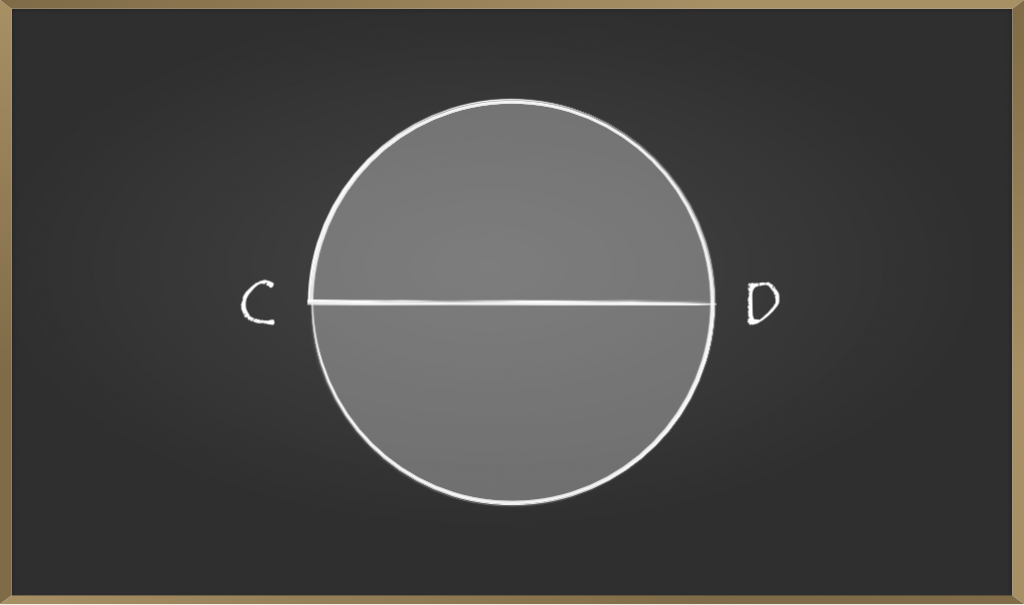• From the figure, the CD is the chord of the circle
• The chord touches the two points in the circle, the two points are CD from above.
• The chord lies within the circle.

Radius is perpendicular to the tangent line

Theorem

The Tangent at any point of a circle is perpendicular to the radius.

Proof

To prove: OP perpendicular to AB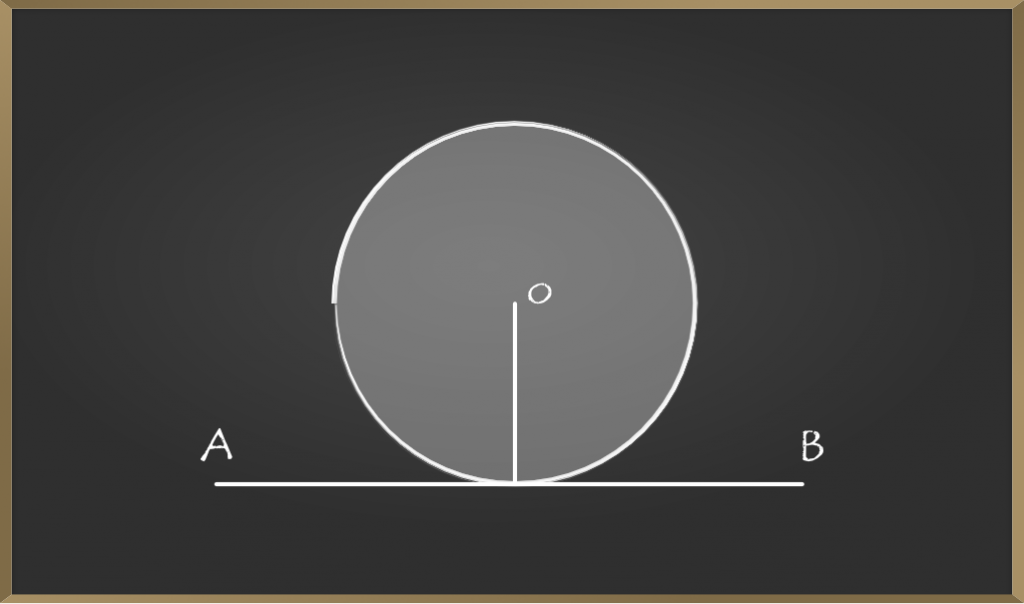• Here O is the center of the circle.
• AB is the tangent to the circle with the center O.
• P is the point of tangency.
• Let Q be a point on the tangent AB.
• Draw an imaginary line from point O to Q it touches the circle at R.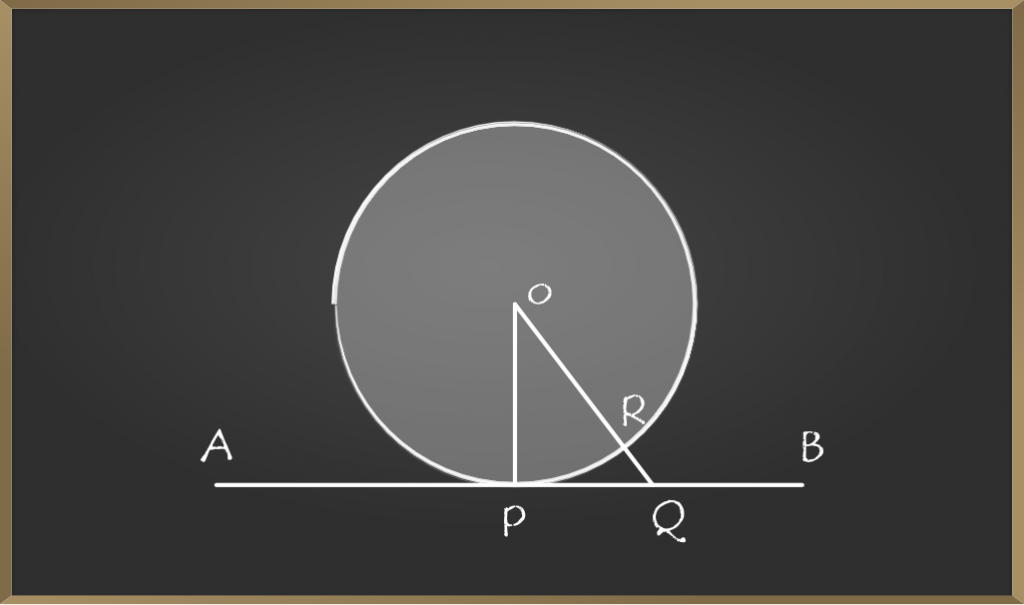• As OQ > OP
• OQ = OR + RQ.
• OP < OR + RQ.
• So same will be the case with all other points on the tangent
• Hence, OP is the smallest line that connects tangent AB.
• We know that the smallest line is always perpendicular. Therefore, OP is perpendicular to AB

Sample Problems on Tangent to a Circle

Problem 1: RA and RB are two tangents to the circle with a radius of 6 cm. Find the length of the arc ACB?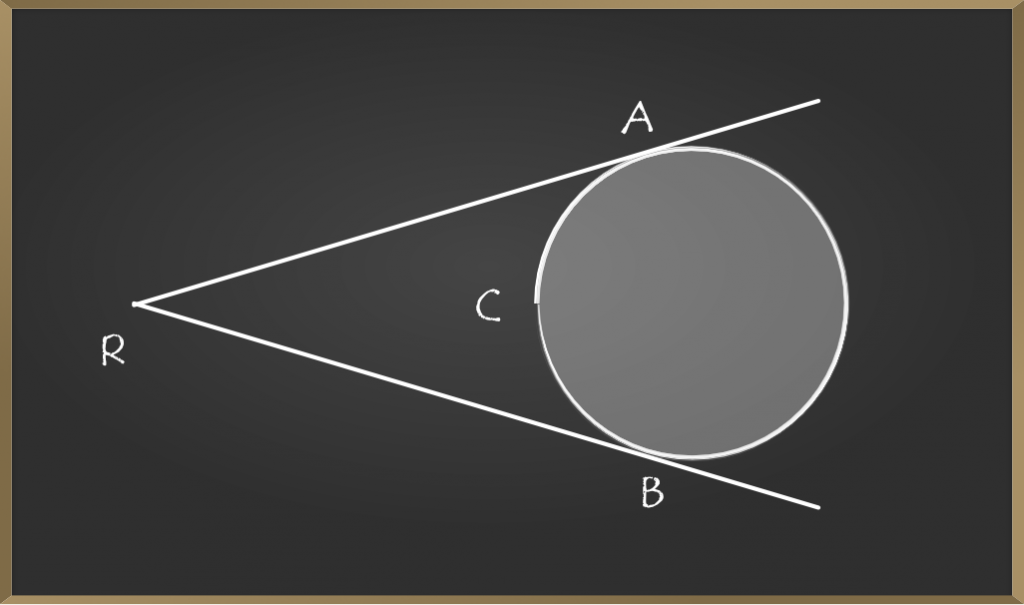Solution:

Step 1: Write all the given values in the question.

RA and RB are two tangents.

Step 2: Write the angle degree between the two tangents RA and RB, if not given the default angle between the two tangents is 60 degrees.

Now the angle between RA and RB is 60 degree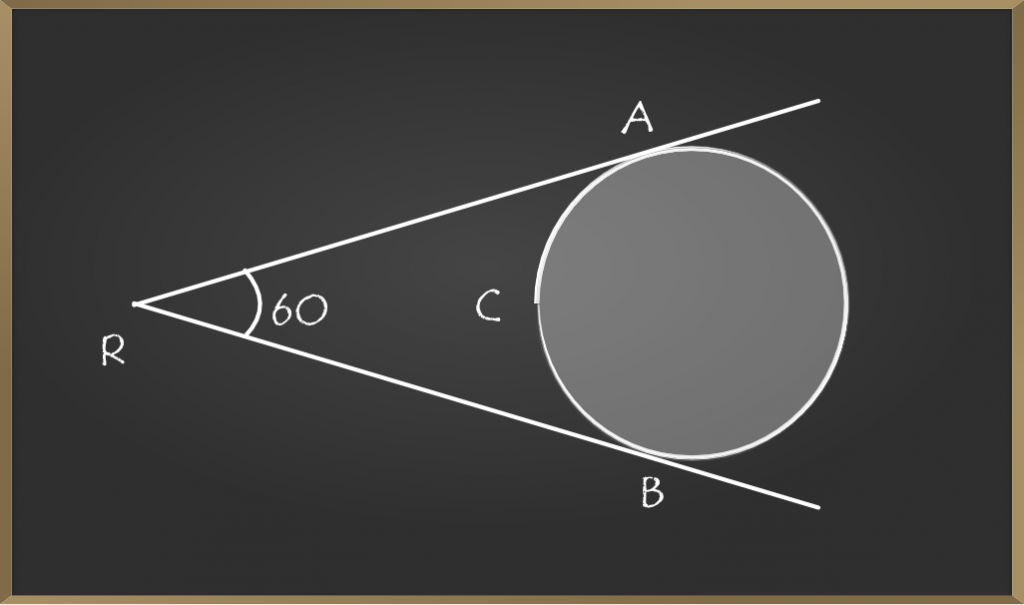Step 3: Try to extend the line from point A to O and B to O it should make 900 with the tangent.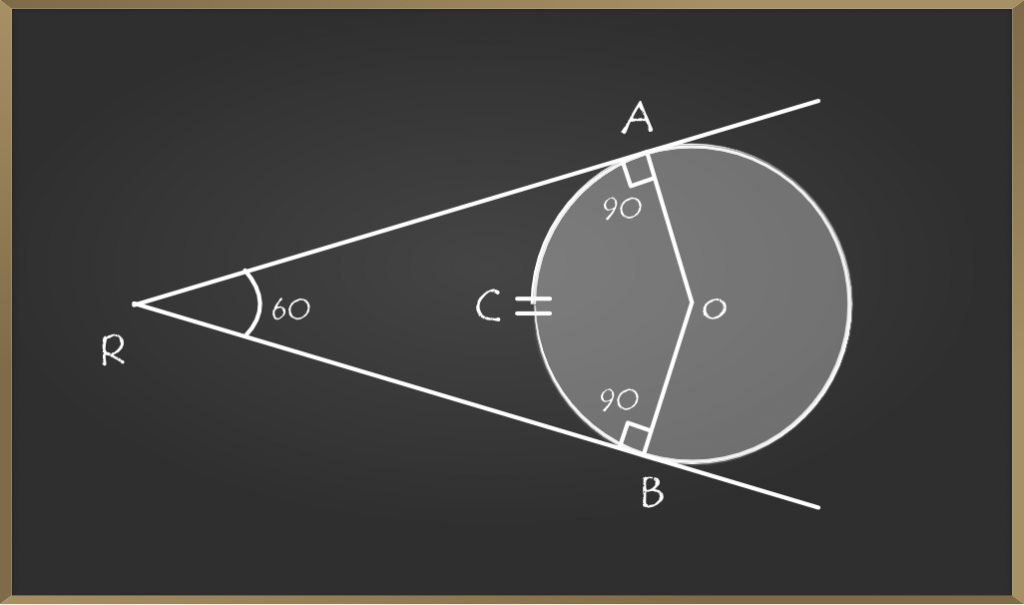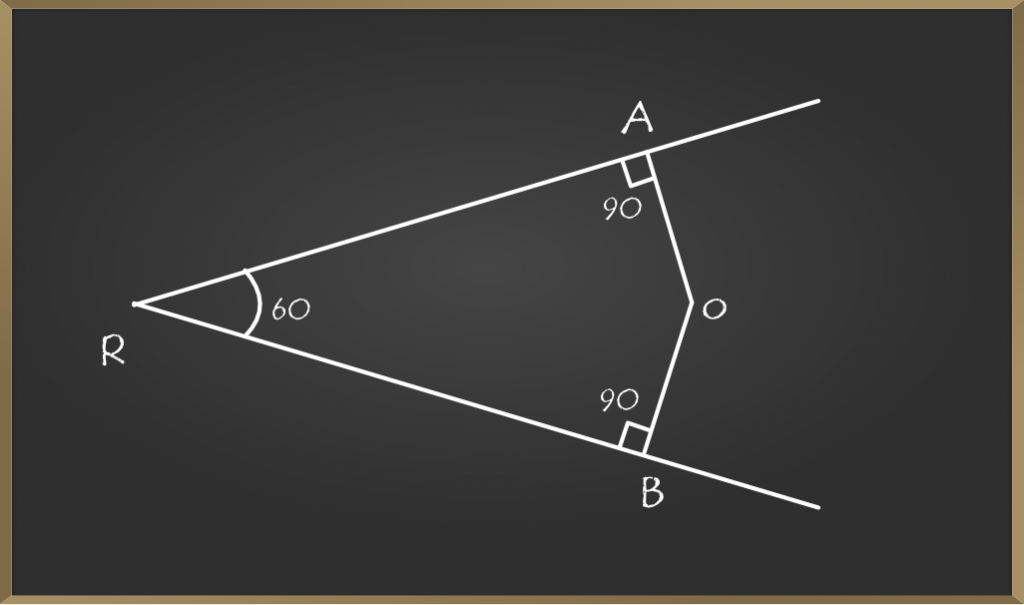Step 4: Apply the rules of a quadrilateral to find the angle between AOB.

here RAOB will be a quadrilateral. So, Ro + Ao + Bo+ AOB = 3600

60o + 90o + 90 + AOB0 = 3600

AOB0 = 3600 – 2400

AOB0 = 1200

Note: Ao = Bo = 90 Since A, B are perpendicular to the tangents RA and RB.

Step 5: Now we need to find the length of ARC by using the following formula

Length of arc = (angle/360) * radius

We get = (120 / 360) * 6

= (1.9) = 2

therefore, the length of the arc ACB is 2 cm.

Problem 2: RA and RB are two tangents to the circle with a radius of 9 cm. Find the length of the arc ACB?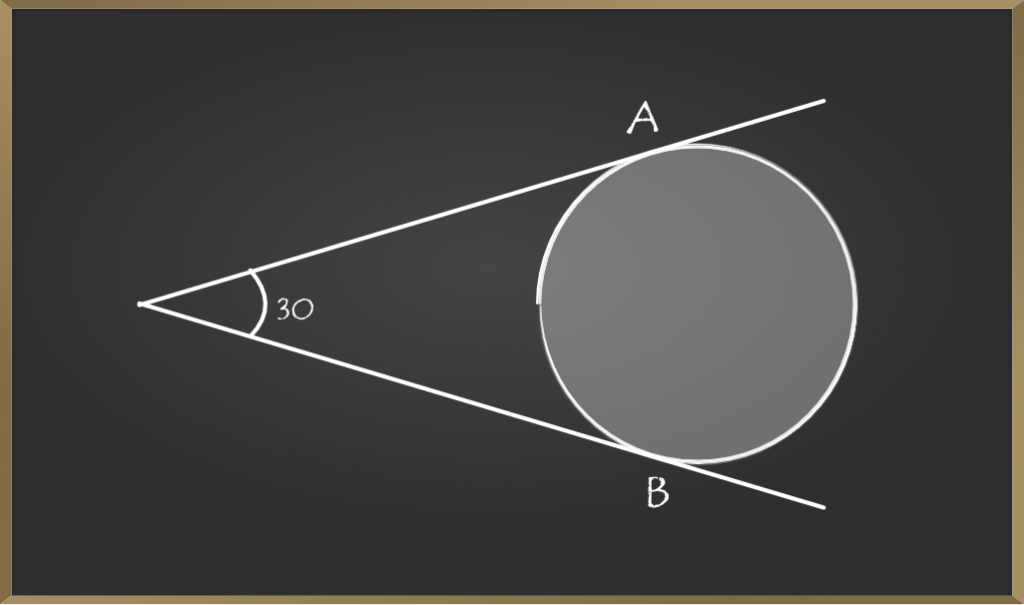Solution:

Given RA and RB are two tangents.

Radius r = 6, lets us assume the point  where two tangent is R

And angle between two tangents RA and RB is 300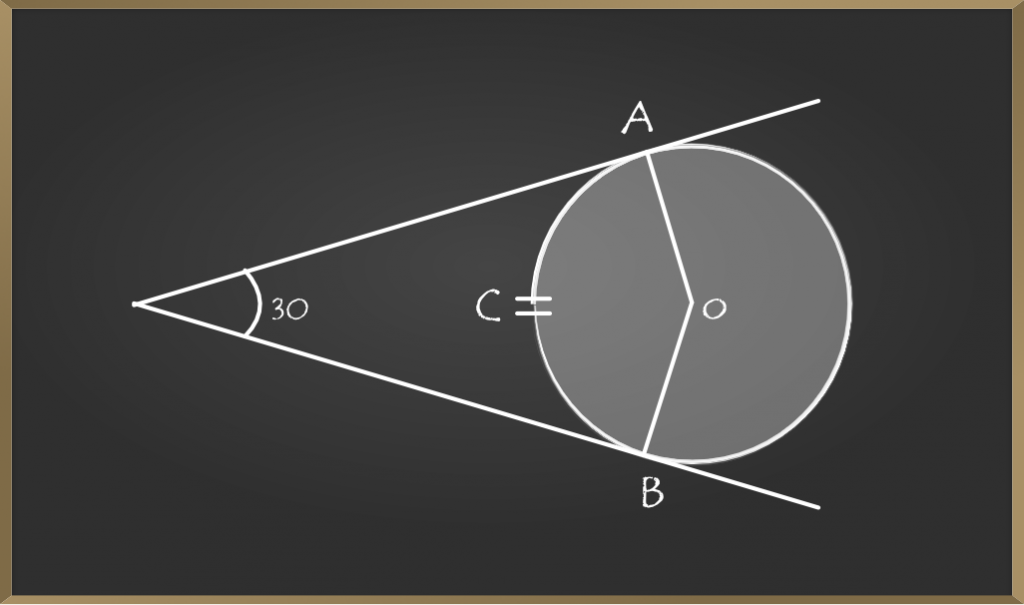Extend the line from point  A to  O and B to O it should make 900 with the tangent.

It forms a quadrilateral as above.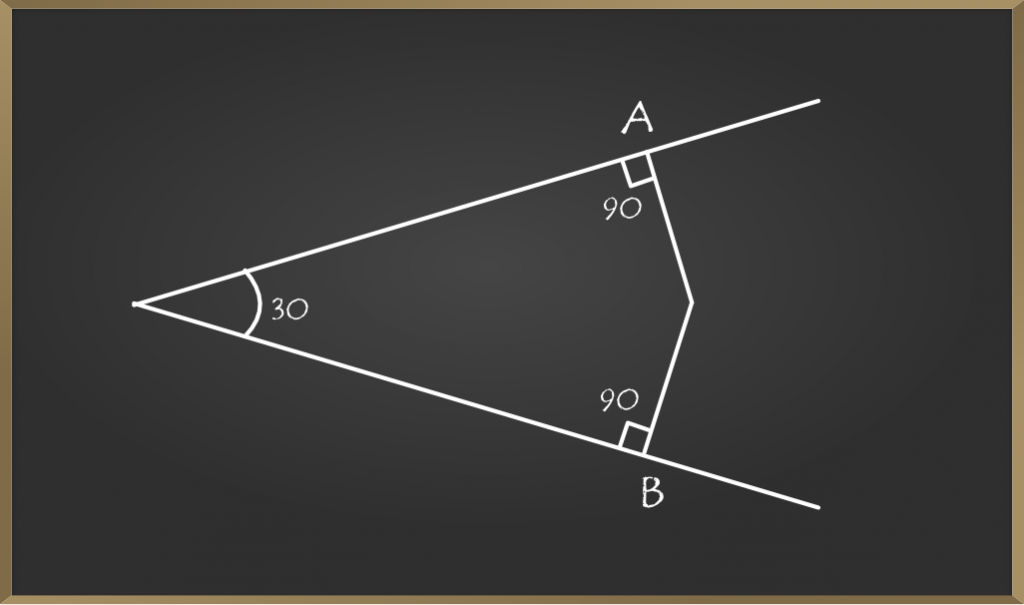Here RAOB will be a quadrilateral So, Ro + Ao + Bo + AOB = 3600.

30o + 90o + 900  + AOB0 = 3600

AOB0 = 3600 – 2100

AOB0 = 1500

Note: Ao = Bo = 90 since A, B are perpendicular to the tangents RA and RB.

Length of arc = (angle/360) * radius

We get = (150 / 360) * 9

= (3.75) = 4cm

Problem 3: Find the value of x from the given figure.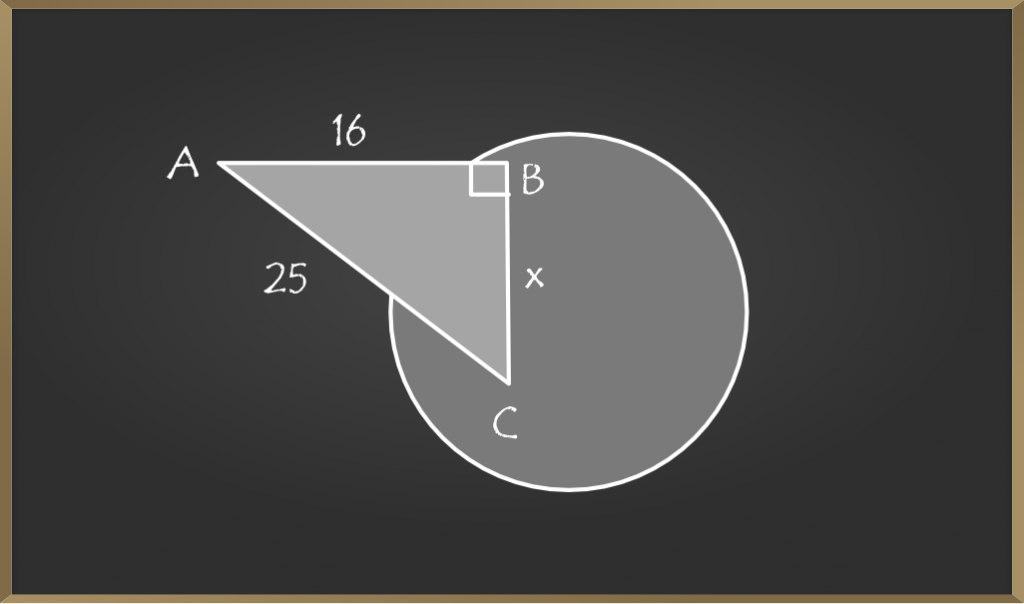Solution

Given A is tangent (from the figure)

by using Right angle triangle properties we can find the value of x

i.e A2 + B2 = C2

A = 16cm, B = xcm, C = 25cm

(16)2 + (x)2 = (25)2

256 +  (x)2  = 625

(x)2 = 625 – 256

(x)2 = 369

Taking root on both the sides

√(x)2 = √369   (square and root get cancelled)

we get, x = 3√41cm.

My Personal Notes arrow_drop_up